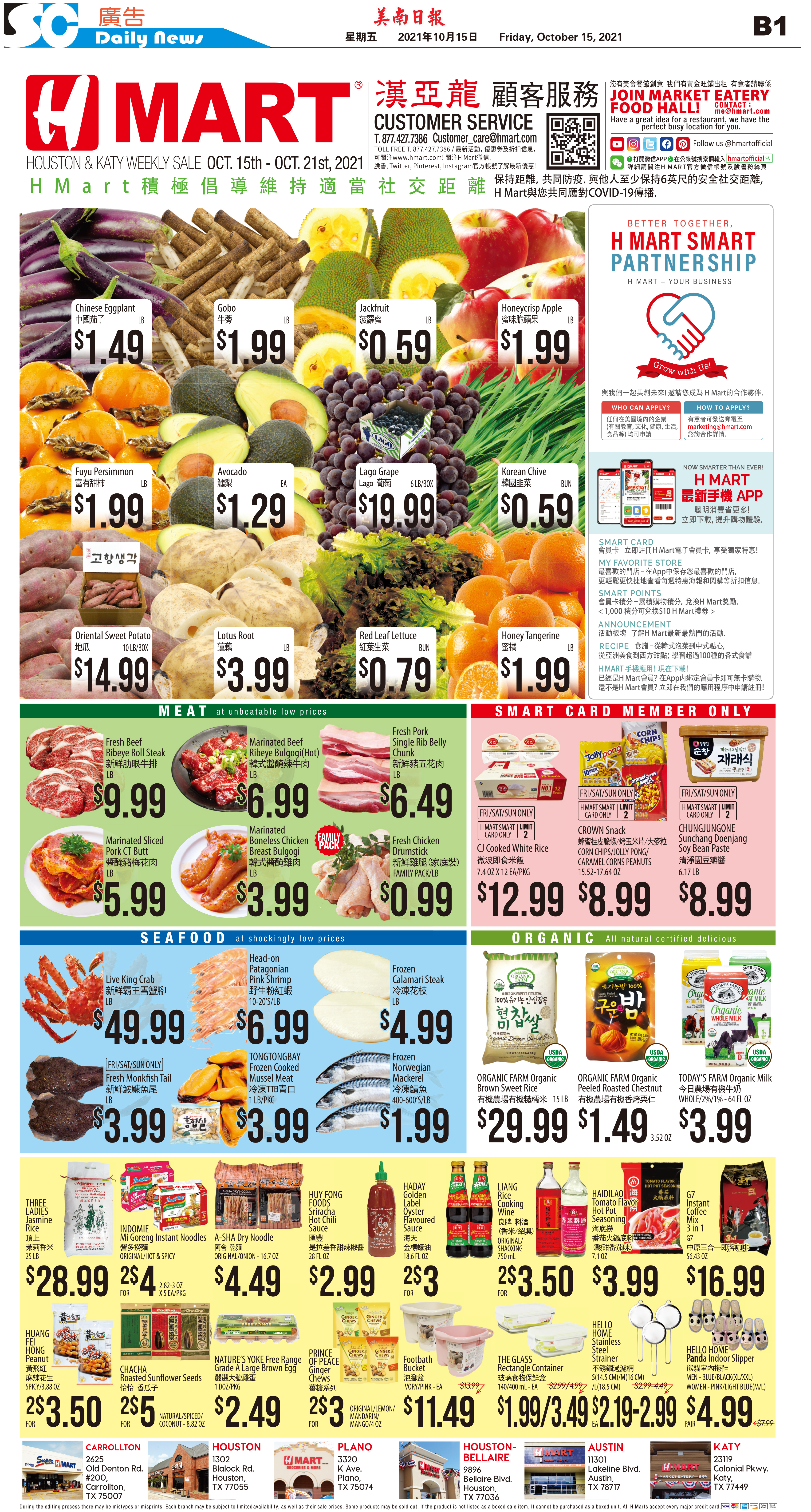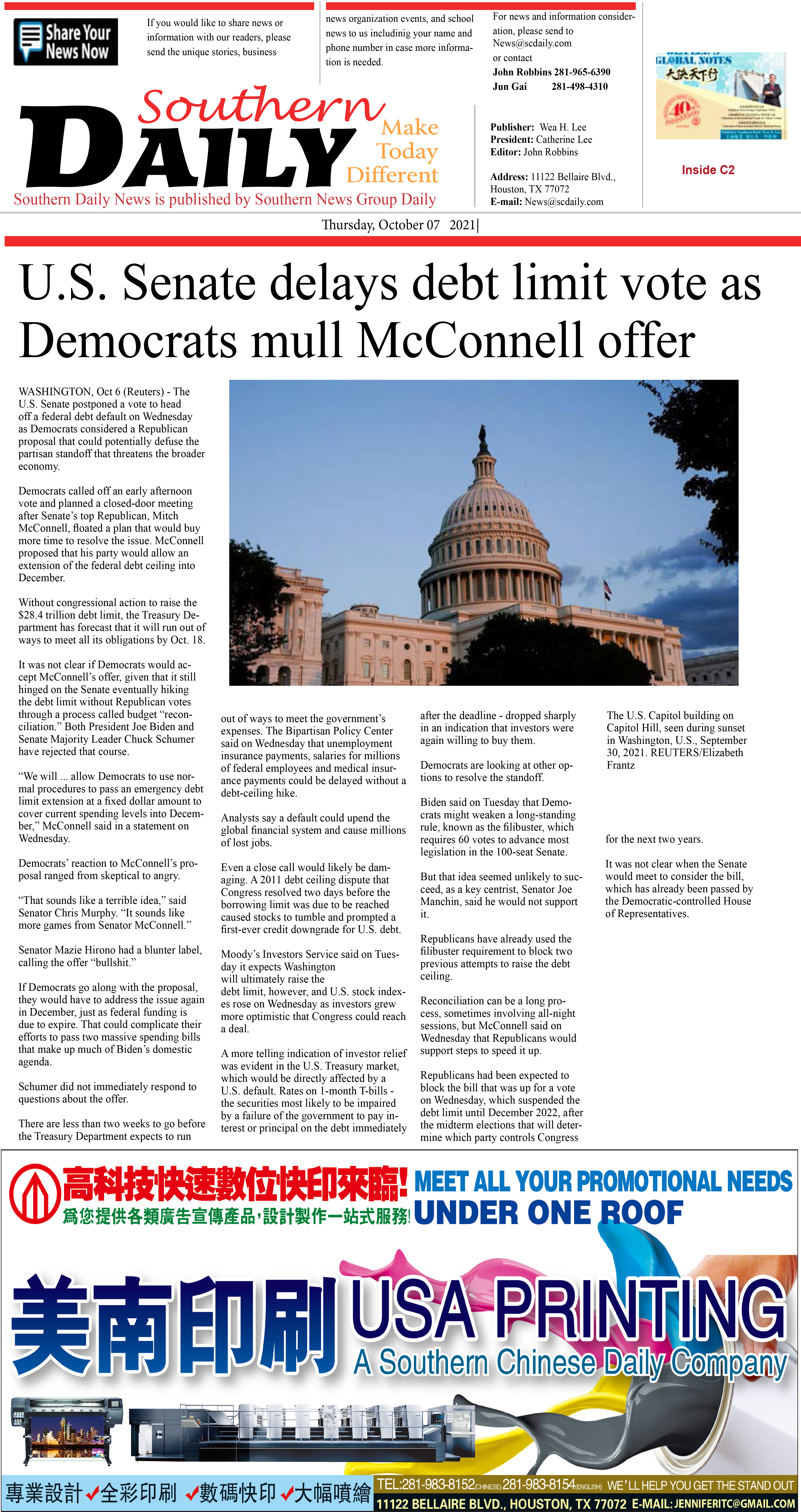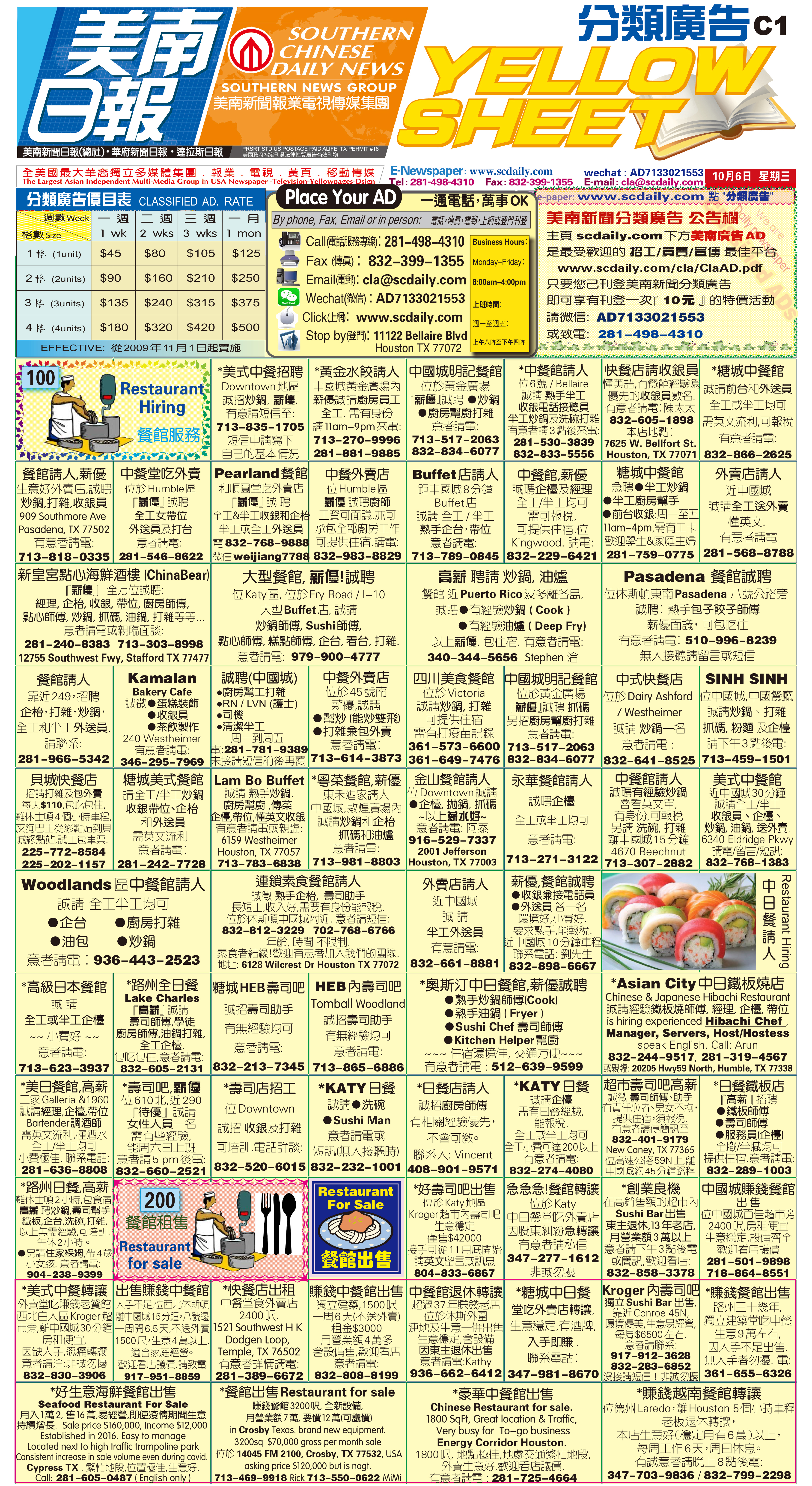211016 Epaper

 A Section B SectionC Section D Section211015 Epaper

 A Section B SectionC Section D Section211014 Epaper

 A Section B SectionC Section D Section211013 Epaper

 A Section B SectionC Section D Section211012 Epaper

 A Section B SectionC Section D Section211011 Epaper

 A Section B SectionC Section D Section211010 Epaper

 A Section B SectionC Section D Section211009 Epaper

 A Section B SectionC Section D Section211007 Epaper

 A Section B SectionC Section D Section211008 Epaper

 A Section B SectionC Section D Section211006 Epaper

 A Section B SectionC Section D Section